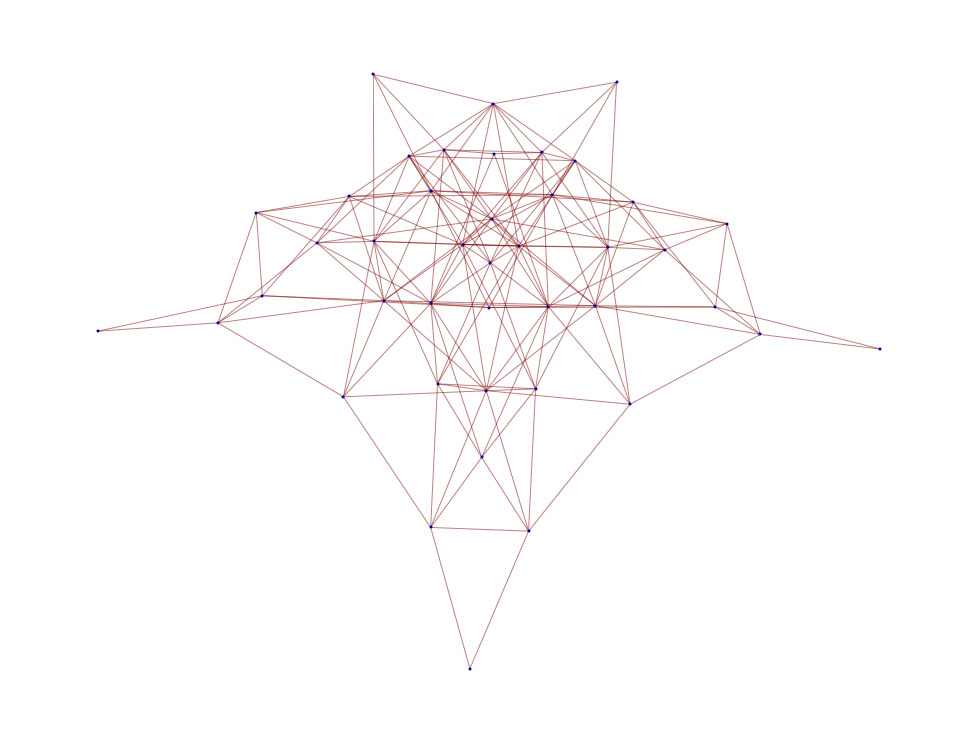# Fusion graphs

 Step 1: Choose a Dynkin diagram G Step 2: Select a positive integer k (called “level”) among those that are proposed Look at the corresponding fusion graphs (there is one graph for each integrable fundamental representations of G at that level) What is this all about? Read the Comments section# Graphs for quantum subgroups Work In Progress

 Step 1: Choose a Dynkin diagram G Step 2: Select a positive integer k (called “level”) among those that are proposed Step 3: Select a quantum subgroup among those that are proposed Look at the corresponding graphs (there is one graph for each integrable fundamental representations of G at that level) What is this all about? Read the Comments section

# Graphs for quantum modules (that are not quantum subgroups) Work In Progress

 Step 1: Choose a Dynkin diagram G Step 2: Select a positive integer k (called “level”) among those that are proposed Step 3: Select a quantum module among those that are proposed Look at the corresponding graphs (there is one graph for each integrable fundamental representations of G at that level) What is this all about? Read the Comments section